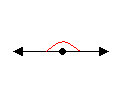# Angles

An angle is the union of two rays with a common endpoint. That endpoint is called the vertex of the angle.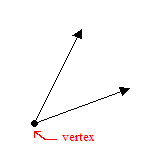An angle is named using the names of three points; one point on each ray and the vertex. Or , if there is no possibility of confusion, you can just use the vertex.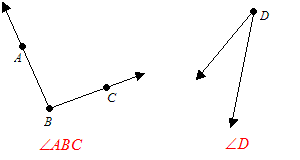(Note that when three points are used, the vertex should go in the middle. So, for example,

$\angle BAC$ would not be a correct name for the angle on the left.)

An angle can be measured by the amount of rotation about the vertex needed for one side to overlap the other. Like rotations, angles can be measured in degrees , where $360$ degrees means one full rotation.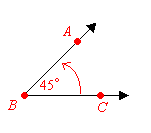In the above figure, $m\angle ABC=45°$ .

Angles can also be measured in units called radians , where $2\pi$ radians is equivalent to $360$ degrees.

## Types of Angles

 Name Measure Example Acute greater than $0°$ , less than $90°$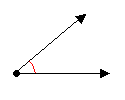Right exactly $90°$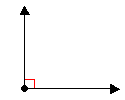Obtuse greater than $90°$ , less than $180°$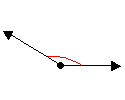Straight exactly $180°$